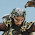## Thursday, 3 January 2013

### Probability for Wargamers 6 - rolling two dice

Moving on, as promised a while back, to rolling two dice. The first question is, what are the odds of rolling any given number on two dice?

Let's demonstrate this empirically, with a large pile of dice (and the help of a bit of image editing, since I don't HAVE 36 purple dice!), to see how many ways there are of rolling a given number. We're using two different colour dice so we can clearly show that (for example) there are three ways of rolling 4.

There are 36 different possible rolls, so (for example) the odds of getting exactly 5 are 4/36, or 1/9, or about 11%.

However, most systems that use 2d6 (WAB, Hail Caesar, BKC, I'm looking at you) tend to require you to roll equal to or under a given number for leadership tests. So we'll be much more interested in what the chances are of, say, rolling 9 or less.

Easy enough - it's the sum (as they're independent events) of the chances of rolling every number 9 or under (or you could just be lazy and count the rolls in the picture). From which we can work out that the chance of rolling 9 or less is (1 + 2 + 3 + 4 + 5 + 6 + 5 + 4) / 36. which happens to be 30/36, or 83%. Scaring up a +1 on your roll from somewhere increases the odds to 33/36, or about 91%. I'll leave drawing up the rest of the table as an exercise for the reader (I always wanted to say that!)

Now to satisfy my curiosity - I have in the past in both BKC and Hail Caesar had commands which flat out refused to activate despite my best efforts with the dice. Let's abstract this away into a general problem. What's the chance of only activating one time in six on a 9 or less?

So - success on a 9 or less is, as we said, 5/6: failure is therefore, obviously enough, 1/6. So if we pass once, and fail five times, the odds are 5/6 * 1/6 * 1/6 * 1/6 * 1/6 * 1/6. Wait a moment, however. That's the probability of succeeding on the first roll and failing on the rest. There are obviously six possible ways to do this, depending on which of the six rolls you succeed on, and each of these are independent, so we can safely add the (identical) probability of each individual way together.

The probability thus works out as (5/6 * 1/6 * 1/6 * 1/6 * 1/6 * 1/6) * 6, or 5/7776.

Wow.

That's 0.06%, or roughly one chance in 1500.

My dice really do suck.

[Performing a similar calculation for an 8 or less roll gets us (26/36 * 10/36 * 10/36 * 10/36 * 10/36 * 10/36) * 6, which works out as 0.7% or one chance in 140. Still sucky.]

1.2.